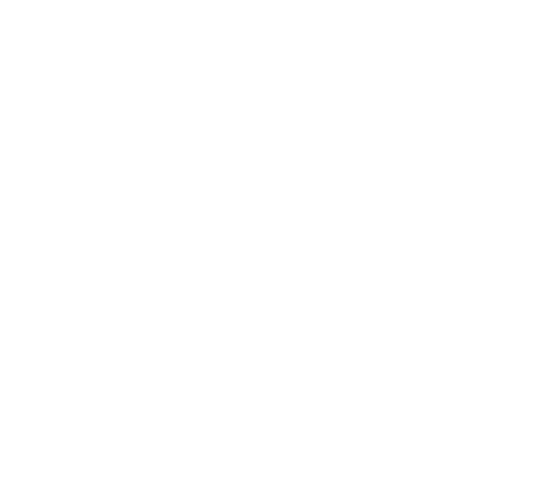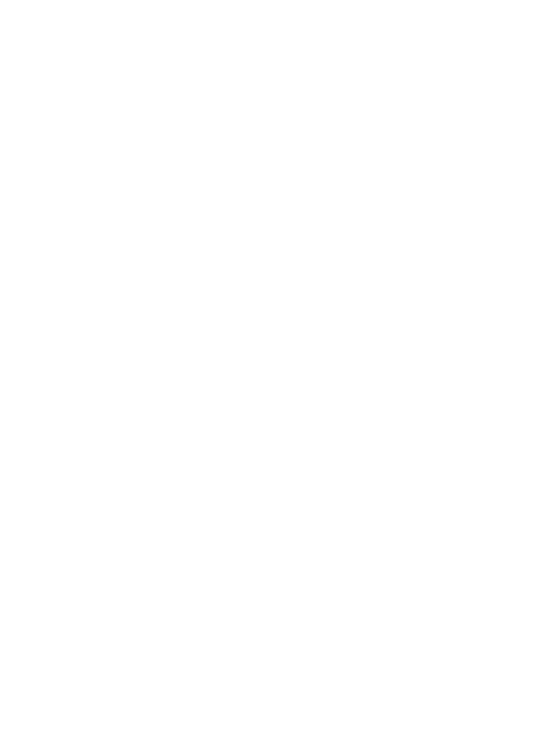### 01. 准备工作

#### 第二步：加载并显示示例图像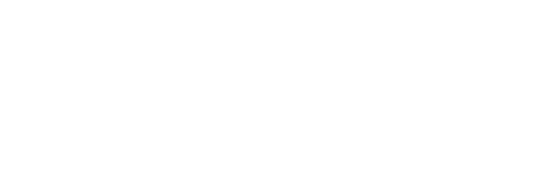### 02. 常用方法

#### 方法一：平均值

```img_temp = img.copy()
img_temp[:,:,0], img_temp[:,:,1], img_temp[:,:,2] = np.average(img, axis=(0,1))

img_temp_2 = img_2.copy()
img_temp_2[:,:,0], img_temp_2[:,:,1], img_temp_2[:,:,2] = np.average(img_2, axis=(0,1))

show_img_compar(img, img_temp)
show_img_compar(img_2, img_temp_2)```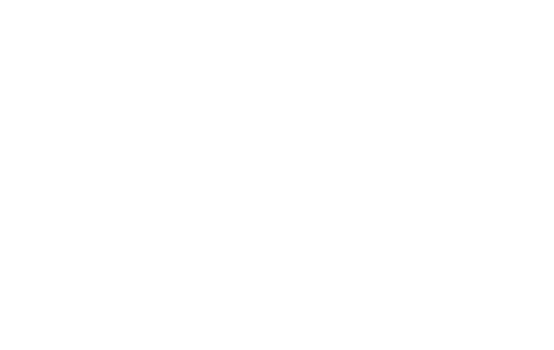#### 方法二：最高像素频率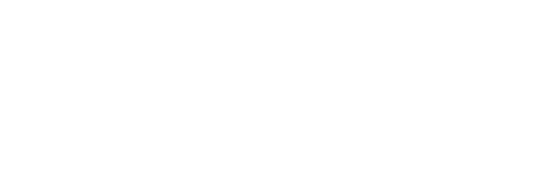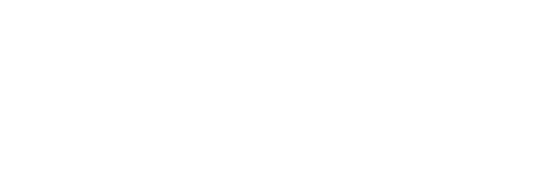```img_temp = img.copy()
unique, counts = np.unique(img_temp.reshape(-1, 3), axis=0, return_counts=True)
img_temp[:,:,0], img_temp[:,:,1], img_temp[:,:,2] = unique[np.argmax(counts)]

img_temp_2 = img_2.copy()
unique, counts = np.unique(img_temp_2.reshape(-1, 3), axis=0, return_counts=True)
img_temp_2[:,:,0], img_temp_2[:,:,1], img_temp_2[:,:,2] = unique[np.argmax(counts)]

show_img_compar(img, img_temp)
show_img_compar(img_2, img_temp_2)```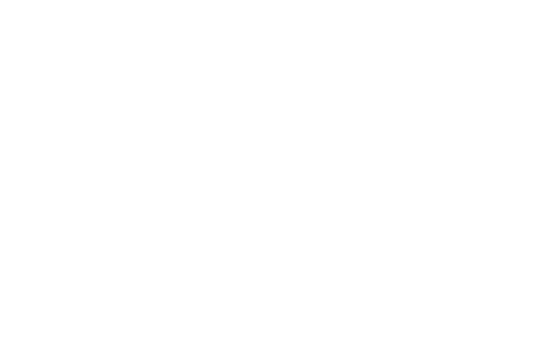#### 方法三：使用K均值聚类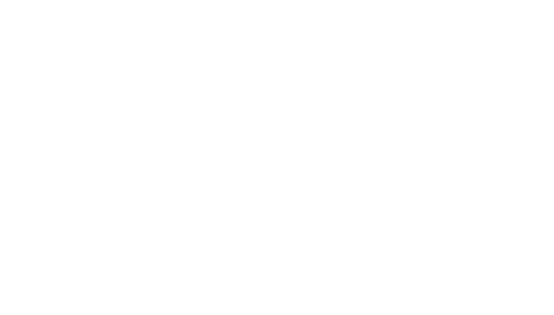```def palette(clusters):
width=300
palette = np.zeros((50, width, 3), np.uint8)
steps = width/clusters.cluster_centers_.shape
for idx, centers in enumerate(clusters.cluster_centers_):
palette[:, int(idx*steps):(int((idx+1)*steps)), :] = centers
return palette

clt_1 = clt.fit(img.reshape(-1, 3))
show_img_compar(img, palette(clt_1))

clt_2 = clt.fit(img_2.reshape(-1, 3))
show_img_compar(img_2, palette(clt_2))```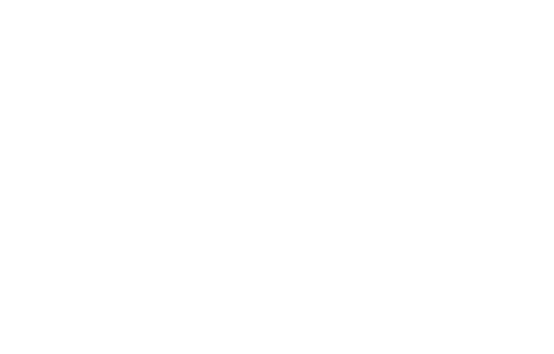```def palette(clusters):
width=300
palette = np.zeros((50, width, 3), np.uint8)
steps = width/clusters.cluster_centers_.shape
for idx, centers in enumerate(clusters.cluster_centers_):
palette[:, int(idx*steps):(int((idx+1)*steps)), :] = centers
return palette

clt_3 = KMeans(n_clusters=3)
clt_3.fit(img_2.reshape(-1, 3))
show_img_compar(img_2, palette(clt_3))```#### 方法四：K均值+比例显示

```from collections import Counter

def palette_perc(k_cluster):
width = 300
palette = np.zeros((50, width, 3), np.uint8)

n_pixels = len(k_cluster.labels_)
counter = Counter(k_cluster.labels_) # count how many pixels per cluster
perc = {}
for i in counter:
perc[i] = np.round(counter[i]/n_pixels, 2)
perc = dict(sorted(perc.items()))

#for logging purposes
print(perc)
print(k_cluster.cluster_centers_)

step = 0

for idx, centers in enumerate(k_cluster.cluster_centers_):
palette[:, step:int(step + perc[idx]*width+1), :] = centers
step += int(perc[idx]*width+1)

return palette

clt_1 = clt.fit(img.reshape(-1, 3))
show_img_compar(img, palette_perc(clt_1))

clt_2 = clt.fit(img_2.reshape(-1, 3))
show_img_compar(img_2, palette_perc(clt_2))```